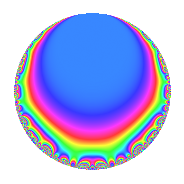# Properties

 Label 57.2.e.aLevel 57 Weight 2 Character orbit 57.e Analytic conductor 0.455 Analytic rank 0 Dimension 2 CM No Inner twists 2

# Related objects

## Newspace parameters

 Level: $$N$$ = $$57 = 3 \cdot 19$$ Weight: $$k$$ = $$2$$ Character orbit: $$[\chi]$$ = 57.e (of order $$3$$ and degree $$2$$)

## Newform invariants

 Self dual: No Analytic conductor: $$0.455147291521$$ Analytic rank: $$0$$ Dimension: $$2$$ Coefficient field: $$\Q(\sqrt{-3})$$ Coefficient ring: $$\Z[a_1, a_2]$$ Coefficient ring index: $$1$$ Sato-Tate group: $\mathrm{SU}(2)[C_{3}]$

## $q$-expansion

Coefficients of the $$q$$-expansion are expressed in terms of a primitive root of unity $$\zeta_{6}$$. We also show the integral $$q$$-expansion of the trace form.

 $$f(q)$$ $$=$$ $$q$$ $$+ ( -1 + \zeta_{6} ) q^{2}$$ $$+ ( 1 - \zeta_{6} ) q^{3}$$ $$+ \zeta_{6} q^{4}$$ $$+ \zeta_{6} q^{6}$$ $$+ q^{7}$$ $$-3 q^{8}$$ $$-\zeta_{6} q^{9}$$ $$+O(q^{10})$$ $$q$$ $$+ ( -1 + \zeta_{6} ) q^{2}$$ $$+ ( 1 - \zeta_{6} ) q^{3}$$ $$+ \zeta_{6} q^{4}$$ $$+ \zeta_{6} q^{6}$$ $$+ q^{7}$$ $$-3 q^{8}$$ $$-\zeta_{6} q^{9}$$ $$-2 q^{11}$$ $$+ q^{12}$$ $$-5 \zeta_{6} q^{13}$$ $$+ ( -1 + \zeta_{6} ) q^{14}$$ $$+ ( 1 - \zeta_{6} ) q^{16}$$ $$+ ( 4 - 4 \zeta_{6} ) q^{17}$$ $$+ q^{18}$$ $$+ ( -5 + 2 \zeta_{6} ) q^{19}$$ $$+ ( 1 - \zeta_{6} ) q^{21}$$ $$+ ( 2 - 2 \zeta_{6} ) q^{22}$$ $$+ 4 \zeta_{6} q^{23}$$ $$+ ( -3 + 3 \zeta_{6} ) q^{24}$$ $$+ 5 \zeta_{6} q^{25}$$ $$+ 5 q^{26}$$ $$- q^{27}$$ $$+ \zeta_{6} q^{28}$$ $$+ 8 \zeta_{6} q^{29}$$ $$-3 q^{31}$$ $$-5 \zeta_{6} q^{32}$$ $$+ ( -2 + 2 \zeta_{6} ) q^{33}$$ $$+ 4 \zeta_{6} q^{34}$$ $$+ ( 1 - \zeta_{6} ) q^{36}$$ $$+ 3 q^{37}$$ $$+ ( 3 - 5 \zeta_{6} ) q^{38}$$ $$-5 q^{39}$$ $$+ ( 12 - 12 \zeta_{6} ) q^{41}$$ $$+ \zeta_{6} q^{42}$$ $$+ ( 1 - \zeta_{6} ) q^{43}$$ $$-2 \zeta_{6} q^{44}$$ $$-4 q^{46}$$ $$+ 6 \zeta_{6} q^{47}$$ $$-\zeta_{6} q^{48}$$ $$-6 q^{49}$$ $$-5 q^{50}$$ $$-4 \zeta_{6} q^{51}$$ $$+ ( 5 - 5 \zeta_{6} ) q^{52}$$ $$-4 \zeta_{6} q^{53}$$ $$+ ( 1 - \zeta_{6} ) q^{54}$$ $$-3 q^{56}$$ $$+ ( -3 + 5 \zeta_{6} ) q^{57}$$ $$-8 q^{58}$$ $$+ ( -10 + 10 \zeta_{6} ) q^{59}$$ $$+ 13 \zeta_{6} q^{61}$$ $$+ ( 3 - 3 \zeta_{6} ) q^{62}$$ $$-\zeta_{6} q^{63}$$ $$+ 7 q^{64}$$ $$-2 \zeta_{6} q^{66}$$ $$-11 \zeta_{6} q^{67}$$ $$+ 4 q^{68}$$ $$+ 4 q^{69}$$ $$+ ( -6 + 6 \zeta_{6} ) q^{71}$$ $$+ 3 \zeta_{6} q^{72}$$ $$+ ( 11 - 11 \zeta_{6} ) q^{73}$$ $$+ ( -3 + 3 \zeta_{6} ) q^{74}$$ $$+ 5 q^{75}$$ $$+ ( -2 - 3 \zeta_{6} ) q^{76}$$ $$-2 q^{77}$$ $$+ ( 5 - 5 \zeta_{6} ) q^{78}$$ $$+ ( -1 + \zeta_{6} ) q^{79}$$ $$+ ( -1 + \zeta_{6} ) q^{81}$$ $$+ 12 \zeta_{6} q^{82}$$ $$+ q^{84}$$ $$+ \zeta_{6} q^{86}$$ $$+ 8 q^{87}$$ $$+ 6 q^{88}$$ $$+ 6 \zeta_{6} q^{89}$$ $$-5 \zeta_{6} q^{91}$$ $$+ ( -4 + 4 \zeta_{6} ) q^{92}$$ $$+ ( -3 + 3 \zeta_{6} ) q^{93}$$ $$-6 q^{94}$$ $$-5 q^{96}$$ $$+ ( -2 + 2 \zeta_{6} ) q^{97}$$ $$+ ( 6 - 6 \zeta_{6} ) q^{98}$$ $$+ 2 \zeta_{6} q^{99}$$ $$+O(q^{100})$$ $$\operatorname{Tr}(f)(q)$$ $$=$$ $$2q$$ $$\mathstrut -\mathstrut q^{2}$$ $$\mathstrut +\mathstrut q^{3}$$ $$\mathstrut +\mathstrut q^{4}$$ $$\mathstrut +\mathstrut q^{6}$$ $$\mathstrut +\mathstrut 2q^{7}$$ $$\mathstrut -\mathstrut 6q^{8}$$ $$\mathstrut -\mathstrut q^{9}$$ $$\mathstrut +\mathstrut O(q^{10})$$ $$2q$$ $$\mathstrut -\mathstrut q^{2}$$ $$\mathstrut +\mathstrut q^{3}$$ $$\mathstrut +\mathstrut q^{4}$$ $$\mathstrut +\mathstrut q^{6}$$ $$\mathstrut +\mathstrut 2q^{7}$$ $$\mathstrut -\mathstrut 6q^{8}$$ $$\mathstrut -\mathstrut q^{9}$$ $$\mathstrut -\mathstrut 4q^{11}$$ $$\mathstrut +\mathstrut 2q^{12}$$ $$\mathstrut -\mathstrut 5q^{13}$$ $$\mathstrut -\mathstrut q^{14}$$ $$\mathstrut +\mathstrut q^{16}$$ $$\mathstrut +\mathstrut 4q^{17}$$ $$\mathstrut +\mathstrut 2q^{18}$$ $$\mathstrut -\mathstrut 8q^{19}$$ $$\mathstrut +\mathstrut q^{21}$$ $$\mathstrut +\mathstrut 2q^{22}$$ $$\mathstrut +\mathstrut 4q^{23}$$ $$\mathstrut -\mathstrut 3q^{24}$$ $$\mathstrut +\mathstrut 5q^{25}$$ $$\mathstrut +\mathstrut 10q^{26}$$ $$\mathstrut -\mathstrut 2q^{27}$$ $$\mathstrut +\mathstrut q^{28}$$ $$\mathstrut +\mathstrut 8q^{29}$$ $$\mathstrut -\mathstrut 6q^{31}$$ $$\mathstrut -\mathstrut 5q^{32}$$ $$\mathstrut -\mathstrut 2q^{33}$$ $$\mathstrut +\mathstrut 4q^{34}$$ $$\mathstrut +\mathstrut q^{36}$$ $$\mathstrut +\mathstrut 6q^{37}$$ $$\mathstrut +\mathstrut q^{38}$$ $$\mathstrut -\mathstrut 10q^{39}$$ $$\mathstrut +\mathstrut 12q^{41}$$ $$\mathstrut +\mathstrut q^{42}$$ $$\mathstrut +\mathstrut q^{43}$$ $$\mathstrut -\mathstrut 2q^{44}$$ $$\mathstrut -\mathstrut 8q^{46}$$ $$\mathstrut +\mathstrut 6q^{47}$$ $$\mathstrut -\mathstrut q^{48}$$ $$\mathstrut -\mathstrut 12q^{49}$$ $$\mathstrut -\mathstrut 10q^{50}$$ $$\mathstrut -\mathstrut 4q^{51}$$ $$\mathstrut +\mathstrut 5q^{52}$$ $$\mathstrut -\mathstrut 4q^{53}$$ $$\mathstrut +\mathstrut q^{54}$$ $$\mathstrut -\mathstrut 6q^{56}$$ $$\mathstrut -\mathstrut q^{57}$$ $$\mathstrut -\mathstrut 16q^{58}$$ $$\mathstrut -\mathstrut 10q^{59}$$ $$\mathstrut +\mathstrut 13q^{61}$$ $$\mathstrut +\mathstrut 3q^{62}$$ $$\mathstrut -\mathstrut q^{63}$$ $$\mathstrut +\mathstrut 14q^{64}$$ $$\mathstrut -\mathstrut 2q^{66}$$ $$\mathstrut -\mathstrut 11q^{67}$$ $$\mathstrut +\mathstrut 8q^{68}$$ $$\mathstrut +\mathstrut 8q^{69}$$ $$\mathstrut -\mathstrut 6q^{71}$$ $$\mathstrut +\mathstrut 3q^{72}$$ $$\mathstrut +\mathstrut 11q^{73}$$ $$\mathstrut -\mathstrut 3q^{74}$$ $$\mathstrut +\mathstrut 10q^{75}$$ $$\mathstrut -\mathstrut 7q^{76}$$ $$\mathstrut -\mathstrut 4q^{77}$$ $$\mathstrut +\mathstrut 5q^{78}$$ $$\mathstrut -\mathstrut q^{79}$$ $$\mathstrut -\mathstrut q^{81}$$ $$\mathstrut +\mathstrut 12q^{82}$$ $$\mathstrut +\mathstrut 2q^{84}$$ $$\mathstrut +\mathstrut q^{86}$$ $$\mathstrut +\mathstrut 16q^{87}$$ $$\mathstrut +\mathstrut 12q^{88}$$ $$\mathstrut +\mathstrut 6q^{89}$$ $$\mathstrut -\mathstrut 5q^{91}$$ $$\mathstrut -\mathstrut 4q^{92}$$ $$\mathstrut -\mathstrut 3q^{93}$$ $$\mathstrut -\mathstrut 12q^{94}$$ $$\mathstrut -\mathstrut 10q^{96}$$ $$\mathstrut -\mathstrut 2q^{97}$$ $$\mathstrut +\mathstrut 6q^{98}$$ $$\mathstrut +\mathstrut 2q^{99}$$ $$\mathstrut +\mathstrut O(q^{100})$$

## Character Values

We give the values of $$\chi$$ on generators for $$\left(\mathbb{Z}/57\mathbb{Z}\right)^\times$$.

 $$n$$ $$20$$ $$40$$ $$\chi(n)$$ $$1$$ $$-\zeta_{6}$$

## Embeddings

For each embedding $$\iota_m$$ of the coefficient field, the values $$\iota_m(a_n)$$ are shown below.

For more information on an embedded modular form you can click on its label.

Label $$\iota_m(\nu)$$ $$a_{2}$$ $$a_{3}$$ $$a_{4}$$ $$a_{5}$$ $$a_{6}$$ $$a_{7}$$ $$a_{8}$$ $$a_{9}$$ $$a_{10}$$
7.1
 0.5 − 0.866025i 0.5 + 0.866025i
−0.500000 0.866025i 0.500000 + 0.866025i 0.500000 0.866025i 0 0.500000 0.866025i 1.00000 −3.00000 −0.500000 + 0.866025i 0
49.1 −0.500000 + 0.866025i 0.500000 0.866025i 0.500000 + 0.866025i 0 0.500000 + 0.866025i 1.00000 −3.00000 −0.500000 0.866025i 0
 $$n$$: e.g. 2-40 or 990-1000 Significant digits: Format: Complex embeddings Normalized embeddings Satake parameters Satake angles

## Inner twists

Char. orbit Parity Mult. Self Twist Proved
1.a Even 1 trivial yes
19.c Even 1 yes

## Hecke kernels

This newform can be constructed as the kernel of the linear operator $$T_{2}^{2}$$ $$\mathstrut +\mathstrut T_{2}$$ $$\mathstrut +\mathstrut 1$$ acting on $$S_{2}^{\mathrm{new}}(57, [\chi])$$.